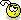## Recommended Posts

here's my attempt at creating an array, I figured I'd create a string of all the die results, then stringsplit that into an array... but the array seems to be empty, or I'm not doing the split right?

```\$XD4 = 5;how many times to roll
Dim \$D4ROLLS[\$XD4]; define array
MsgBox(0, "test", "5d4 test: " & _dieroller(4, \$XD4, \$D4ROLLS))

; \$D is the die to roll, \$X is the number of times to roll it, \$total is the value to return to.
Func _dieroller(\$D, \$X, \$TOTAL)
Do
\$ROLL = Int(Random(\$D) + 1)
\$RESULT = String(\$ROLL & "/")
\$REPS = \$REPS + 1
Until \$REPS = \$X
\$TOTAL = StringSplit(\$RESULT, "/")
Return \$TOTAL
EndFunc  ;==>_dieroller```

"I'm not even supposed to be here today!" -Dante (Hicks)

##### Share on other sites

Untested, but should be close enough.

```Func _dieroller(\$D, \$X, \$TOTAL)
Local \$res = ""
Do
\$ROLL = Int(Random(\$D) + 1)
\$REPS = \$REPS + 1
\$res = \$res & ";" & \$ROLL
Until \$REPS = \$X
\$res = StringTrimLeft(\$res, 1); Leading semi-colon
Local \$a = StringSplit(\$res, ";")
Local \$i, \$t = 0
For \$i = 1 To \$a
\$t = \$t + Number(\$a[\$i])
Next
\$a = \$t
Return \$a
EndFunc ;==>_dieroller```

##### Share on other sites

You can't display an array in a message box since it is not text. Try either returning a string and parsing that how you want, or using some function to display each element of the array (prehaps seperate with a comma-space pair?) Use something like

```Func DisplayArray(\$array) ;returns a string representation of the array
\$return = \$array
For \$i =1 To UBound(\$array) - 1
\$return = \$return & ", " & \$array[\$i]
Next
Return \$return
EndFunc```

Then just concatanate that with what you want in the message box.

[font="Optima"]"Standing in the rain, twisted and insane, we are holding onto nothing.Feeling every breath, holding no regrets, we're still looking out for something."[/font]Note: my projects are off-line until I can spend more time to make them compatable with syntax changes.

##### Share on other sites

my eventual goal with this is to write a GUI die roller, and I figured first step is to setup the function to do the work... I'd like to be able to display two results when someone clicks roll, the individual rolls and the total of those rolls...

I thought that \$array would return the value as the # of lines in the array (why wouldn't that show in a msgbox?), but that doesn't seem to be working for me...

Valik, what does your function return? I'm trying to pick it appart line by line, but I don't think I'm getting it, could you comment it up?

"I'm not even supposed to be here today!" -Dante (Hicks)

##### Share on other sites

I thought that \$array would return the value as the # of lines in the array (why wouldn't that show in a msgbox?), but that doesn't seem to be working for me...

\$array will (from the StringSplit() function) but in your original code you were trying to display "5d4 test: " & _dieroller(4, \$XD4, \$D4ROLLS)

The part in italics (your function call) returns an array, which you cannot put into a message box.

[font="Optima"]"Standing in the rain, twisted and insane, we are holding onto nothing.Feeling every breath, holding no regrets, we're still looking out for something."[/font]Note: my projects are off-line until I can spend more time to make them compatable with syntax changes.

##### Share on other sites

pekster is right.

Here's your code the right way:

```\$XD4 = 5;how many times to roll
Dim \$D4ROLLS[\$XD4]; define array
MsgBox(0, "test", "5d4 test: " & _dieroller(4, \$XD4, \$D4ROLLS))

; \$D is the die to roll, \$X is the number of times to roll it, \$total is the value to return to.
Func _dieroller(\$D, \$X, \$TOTAL)
Do
\$ROLL = Int(Random(\$D) + 1)
\$RESULT = String(\$ROLL & "/")
\$REPS = \$REPS + 1
Until \$REPS = \$X
\$TOTAL = StringSplit(\$RESULT, "/")
Return \$TOTAL
EndFunc ;==>_dieroller```

##### Share on other sites

The function:

```Func _dieroller(\$D, \$X)
Local \$res = "", \$REPS, \$ROLL, \$a, \$i, \$t = 0   ; Declare variables
Do
\$ROLL = Int(Random(\$D) + 1)
\$REPS = \$REPS + 1
\$res = \$res & ";" & \$ROLL   ; Append to string
Until \$REPS = \$X
\$res = StringTrimLeft(\$res, 1); Strip leading semi-colon
\$a = StringSplit(\$res, ";")   ; Split into an array
For \$i = 1 To \$a   ; Loop through the array adding the numbers
\$t = \$t + Number(\$a[\$i])
Next
\$a = \$t   ; Replace element 0 with the total of all the rolls
Return \$a   ; Return the array
EndFunc;==>_dieroller```

The test example:

```Local \$res = _dieroller(6, 10)
MsgBox(4096, "", "Total=" & \$res); Display the total
Local \$i
For \$i = 1 To UBound(\$res) -1; Loop through and display the rest
MsgBox(4096, "", "Roll " & \$i & "=" & \$res[\$i])
Next```

##### Share on other sitesThank you all very much, I didn't intend to ask for it to be done for me, but now that it is, I really appreciate it... I'll keep reading it over and over till I understand all the little parts"I'm not even supposed to be here today!" -Dante (Hicks)

## Create an account

Register a new account

• ### Recently Browsing   0 members

×

• Wiki

• Back

• #### Beta

• Git
• FAQ
• Our Picks
×
• Create New...